### Information

This package contains different models for multi-phase loads.

Extends from Modelica.Icons.Package (Icon for standard packages).

### Package Content

Name Description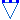WyeLoad_3Ph Three-phase wye load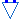WyeLoad_2Ph Two-phase wye load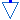WyeLoad_1Ph Single-phase load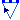WyeDynLoad_3Ph Variable balanced three-phase wye loadDeltaLoad_3Ph Three-phase delta loadDeltaLoad_2Ph Two-phase delta loadDeltaDynLoad_3Ph Variable three-phase delta load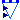Dyn_wye_3Ph_balanced Variable balanced three-phase wye loadDyn_wye_3Ph_unbalanced Variable unbalanced three-phase wye load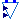Dyn_wye_2Ph_balanced Variable balanced two-phase wye loadDyn_wye_2Ph_unbalanced Variable unbalanced two-phase wye load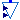Dyn_wye_1Ph Variable single-phase load

##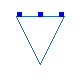OpenIPSL.Electrical.ThreePhase.Loads.WyeLoad_3Ph

### Information

This is a three-phase wye load model.

The user needs to input the active (P_a, P_b and P_c) and reactive (Q_a, Q_b and Q_c) powers consumed by each phase. Since the values come from independent parameters, the model allows the representation of unbalanced loads.

In addition, the model allows the load to be represented as constant power, or as a ZIP model. In the first approach, the powers consumed by each phase are kept constant throughout simulation. The latter approach allows the load to be represented as a sum of three terms: constant impedance, constant current and constant power. This means that, depending on voltage levels supplied, the load will consume different values of power.

Extends from ThreePhaseComponent (Partial model for inheritance of single-phase base power based on three-phase system base power.).

### Parameters

NameDescription
Power flow data
S_bSystem base [V.A]
S_pPhase base [V.A]
VAVoltage magnitude 
AngAVoltage angle for phase A [rad]
VBVoltage magnitude 
AngBVoltage angle for phase B [rad]
VCVoltage magnitude 
AngCVoltage angle for phase C [rad]
P_aInitial active power [W]
Q_aInitial reactive power [var]
P_bInitial active power [W]
Q_bInitial reactive power [var]
P_cInitial active power [W]
Q_cInitial reactive power [var]
Power flow
ModelType0- Constant Power Model, 1- ZIP Model;
A_paPercentage of Constant Power Load for Phase A (%)
B_paPercentage of Constant Current Load for Phase A (%)
C_paPercentage of Constant Impedance Load for Phase A (%)
A_pbPercentage of Constant Power Load for Phase B (%)
B_pbPercentage of Constant Current Load for Phase B (%)
C_pbPercentage of Constant Impedance Load] for Phase B (%)
A_pcPercentage of Constant Power Load for Phase C (%)
B_pcPercentage of Constant Current Load for Phase C (%)
C_pcPercentage of Constant Impedance Load for Phase C (%)

NameDescription
A
B
C

##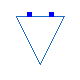OpenIPSL.Electrical.ThreePhase.Loads.WyeLoad_2Ph

### Information

This is a two-phase wye load model.

The user needs to input the active (P_a and P_b) and reactive (Q_a and Q_b) powers consumed by each of the two phases. Since the values come from independent parameters, the model allows the representation of unbalanced loads.

In addition, the model allows the load to be represented as constant power, or as a ZIP model. In the first approach, the powers consumed by each phase are kept constant throughout simulation. The latter approach allows the load to be represented as a sum of three terms: constant impedance, constant current and constant power. This means that, depending on voltage levels supplied, the load will consume different values of power.

Extends from ThreePhaseComponent (Partial model for inheritance of single-phase base power based on three-phase system base power.).

### Parameters

NameDescription
Power flow data
S_bSystem base [V.A]
S_pPhase base [V.A]
Power flow
ModelType0- Constant Power Model, 1- ZIP Model;
P_aActive power for phase A [W]
Q_aReactive power for phase A [var]
P_bActive power for phase B [W]
Q_bReactive power for phase B [var]
Initialization
VAGuess value for phase A magnitude 
AngAGuess value for phase A angle [rad]
VBGuess value for phase B magnitude 
AngBGuess value for phase B angle [rad]
A_paPercentage of Constant Power Load for Phase A [%]
B_paPercentage of Constant Current Load for Phase A [%]
C_paPercentage of Constant Impedance Load for Phase A [%]
A_pbPercentage of Constant Power Load for Phase B [%]
B_pbPercentage of Constant Current Load for Phase B [%]
C_pbPercentage of Constant Impedance Load] for Phase B [%]

NameDescription
A
B

##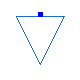OpenIPSL.Electrical.ThreePhase.Loads.WyeLoad_1Ph

### Information

This is a single-phase load model.

The user needs to input the active (P_a) and reactive (Q_a) powers consumed by the phase. Since the values come from independent parameters, the model allows the representation of unbalanced loads.

In addition, the model allows the load to be represented as constant power, or as a ZIP model. In the first approach, the load consumes the same value of active and reactive powers throughout the simulation. The latter approach allows the load to be represented as a sum of three terms: constant impedance, constant current and constant power. This means that, depending on voltage levels supplied, the load will consume different values of power.

Extends from ThreePhaseComponent (Partial model for inheritance of single-phase base power based on three-phase system base power.).

### Parameters

NameDescription
ModelType0- Constant Power Model, 1- ZIP Model;
Power flow data
S_bSystem base [V.A]
S_pPhase base [V.A]
VAVoltage magnitude 
AngAVoltage angle for phase A [rad]
P_aInitial active power [W]
Q_aInitial reactive power [var]
A_paPercentage of Constant Power Load for Phase A [%]
B_paPercentage of Constant Current Load for Phase A [%]
C_paPercentage of Constant Impedance Load for Phase A [%]

NameDescription
A

##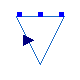OpenIPSL.Electrical.ThreePhase.Loads.WyeDynLoad_3Ph

### Information

This is a three-phase wye load model that allows the user to vary the power consumed during the simulation.

The user needs to input the active (P_a, P_b and P_c) and reactive (Q_a, Q_b and Q_c) powers consumed by each phase. Since the values come from independent parameters, the model allows the representation of unbalanced loads.

In addition, the model allows the load to be represented as constant power, or as a ZIP model. In the first approach, the load consumes the same value of active and reactive powers by each phase throughout the simulation. The latter approach allows the load to be represented as a sum of three terms: constant impedance, constant current and constant power. This means that, depending on voltage levels supplied, the load will consume different values of power.

In addition to that, the real input DynFact multiplies the power being consumed by the load. This allows the load to vary over time during the simulation. It is also important to note that DynFact multiplies all variables P_a, P_b, P_c, Q_a, Q_b and Q_c. That means that this model does not allow load variation to be performed only in selected variables.

Extends from ThreePhaseComponent (Partial model for inheritance of single-phase base power based on three-phase system base power.).

### Parameters

NameDescription
Power flow data
S_bSystem base [V.A]
S_pPhase base [V.A]
Power flow
ModelType0- Constant Power Model, 1- ZIP Model;
P_aBase Active power for phase A [W]
Q_aBase Reactive power for phase A [var]
P_bBase Active power for phase B [W]
Q_bBase Reactive power for phase B [var]
P_cBase Active power for phase C [W]
Q_cBase Reactive power for phase C [var]
A_paPercentage of Constant Power Load for Phase A (%)
B_paPercentage of Constant Current Load for Phase A (%)
C_paPercentage of Constant Impedance Load for Phase A (%)
A_pbPercentage of Constant Power Load for Phase B (%)
B_pbPercentage of Constant Current Load for Phase B (%)
C_pbPercentage of Constant Impedance Load] for Phase B (%)
A_pcPercentage of Constant Power Load for Phase C (%)
B_pcPercentage of Constant Current Load for Phase C (%)
C_pcPercentage of Constant Impedance Load for Phase C (%)

NameDescription
A
B
C

##OpenIPSL.Electrical.ThreePhase.Loads.DeltaLoad_3Ph

### Information

This is a three-phase delta load model.

The user needs to input the active (P_ab, P_bc and P_ca) and reactive (Q_ab, Q_bc and Q_ca) powers consumed between phases. Since the values come from independent parameters, the model allows the representation of unbalanced loads.

In addition, the model allows the load to be represented as constant power, or as a ZIP model. In the first approach, the powers consumed between phases are kept constant throughout simulation. The latter approach allows the load to be represented as a sum of three terms: constant impedance, constant current and constant power. This means that, depending on voltage levels supplied, the load will consume different values of power.

Extends from ThreePhaseComponent (Partial model for inheritance of single-phase base power based on three-phase system base power.).

### Parameters

NameDescription
Power flow data
S_bSystem base [V.A]
S_pPhase base [V.A]
VAVoltage magnitude for phase A 
AngAVoltage angle for phase A [rad]
VBVoltage magnitude for phase B 
AngBVoltage angle for phase B [rad]
VCVoltage magnitude for phase C 
AngCVoltage angle for phase C [rad]
P_abInitial active power [W]
Q_abInitial reactive power [var]
P_bcInitial active power [W]
Q_bcInitial reactive power [var]
P_caInitial active power [W]
Q_caInitial reactive power [var]
Power flow
ModelType0- Constant Power Model, 1- ZIP Model;
A_abPercentage of Constant Power Load for Line AB [%]
B_abPercentage of Constant Current Load for Line AB [%]
C_abPercentage of Constant Impedance Load for Line AB [%]
A_bcPercentage of Constant Power Load for Line BC [%]
B_bcPercentage of Constant Current Load for Line BC [%]
C_bcPercentage of Constant Impedance Load] for Line BC [%]
A_caPercentage of Constant Power Load for Line CA [%]
B_caPercentage of Constant Current Load for Line CA [%]
C_caPercentage of Constant Impedance Load for Line CA [%]

NameDescription
A
B
C

##OpenIPSL.Electrical.ThreePhase.Loads.DeltaLoad_2Ph

### Information

This is a two-phase delta load model. The model allows the user to represent active and reactive power being consumed between two phases.

The user needs to input the active (P_ab) and reactive (Q_ab) powera consumed between phases one and two (A and B).

In addition, the model allows the load to be represented as constant power, or as a ZIP model. In the first approach, the power consumed between the two phases is kept constant throughout simulation. The latter approach allows the load to be represented as a sum of three terms: constant impedance, constant current and constant power. This means that, depending on voltage levels supplied, the load will consume a different power value.

Extends from ThreePhaseComponent (Partial model for inheritance of single-phase base power based on three-phase system base power.).

### Parameters

NameDescription
Power flow data
S_bSystem base [V.A]
S_pPhase base [V.A]
VAVoltage magnitude for phase A 
AngAVoltage angle for phase A [rad]
VBVoltage magnitude for phase B 
AngBVoltage angle for phase B [rad]
P_abInitial active power [W]
Q_abInitial reactive power [var]
Power flow
ModelType0- Constant Power Model, 1- ZIP Model;
A_abPercentage of Constant Power Load for Line AB [%]
B_abPercentage of Constant Current Load for Line AB [%]
C_abPercentage of Constant Impedance Load for Line AB [%]

NameDescription
A
B

##OpenIPSL.Electrical.ThreePhase.Loads.DeltaDynLoad_3Ph

### Information

This is a three-phase delta load model that allows the user to vary the power consumed during the simulation.

The user needs to input the active (P_ab, P_bc and P_ca) and reactive (Q_ab, Q_bc and Q_ca) powers consumed between phases. Since the values come from independent parameters, the model allows the representation of unbalanced loads.

In addition, the model allows the load to be represented as constant power, or as a ZIP model. In the first approach, the load consumes the same value of active and reactive powers between phases throughout the simulation. The latter approach allows the load to be represented as a sum of three terms: constant impedance, constant current and constant power. This means that, depending on voltage levels supplied, the load will consume different values of power.

In addition to that, the real input DynFact multiplies the power being consumed by the load. This allows the load to vary over time during the simulation. It is also important to note that DynFact multiplies all variables P_ab, P_bc, P_ca, Q_ab, Q_bc and Q_ca. That means that this model does not allow load variation to be performed only in selected variables.

Extends from ThreePhaseComponent (Partial model for inheritance of single-phase base power based on three-phase system base power.).

### Parameters

NameDescription
Power flow data
S_bSystem base [V.A]
S_pPhase base [V.A]
Power flow
ModelType0- Constant Power Model, 1- ZIP Model;
P_abActive power for line AB [W]
Q_abReactive power for line AB [var]
P_bcActive power for line BC [W]
Q_bcReactive power for line BC [var]
P_caActive power for line CA [W]
Q_caReactive power for line CA [var]
A_abPercentage of Constant Power Load for Line AB (%)
B_abPercentage of Constant Current Load for Line AB (%)
C_abPercentage of Constant Impedance Load for Line AB (%)
A_bcPercentage of Constant Power Load for Line BC (%)
B_bcPercentage of Constant Current Load for Line BC (%)
C_bcPercentage of Constant Impedance Load] for Line BC (%)
A_caPercentage of Constant Power Load for Line CA (%)
B_caPercentage of Constant Current Load for Line CA (%)
C_caPercentage of Constant Impedance Load for Line CA (%)

NameDescription
A
B
C

##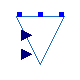OpenIPSL.Electrical.ThreePhase.Loads.Dyn_wye_3Ph_balanced

### Information

This is a three-phase wye load model that allows the user to vary the power consumed during the simulation.

The user needs to input the entire active (P0) and reactive (Q0) powers consumed by the load. Since the model represents a balanced load, the active and reactive powers consumed by each phase are P0/3 and Q0/3. The model allows the load to be represented as constant power only.

In addition to that, the real inputs P_in and Q_in multiply the values of active and reactive power, respectively, being consumed by the load. This allows the load to vary its consumed power over time during the simulation. Note that, since active and reactive powers can vary independently, the load's power factor can also vary. In addition, note that by changing P0 and Q0, the values of power consumed by the three phases vary identically. This makes the load to be characterized as balanced.

Extends from ThreePhaseComponent (Partial model for inheritance of single-phase base power based on three-phase system base power.).

### Parameters

NameDescription
Power flow data
S_bSystem base [V.A]
S_pPhase base [V.A]
Power flow
P0Initial Active power [W]
Q0Initial Reactive power [var]

### Connectors

NameDescription
P_inexternal P [pu]
Q_inexternal Q [pu]
A
B
C

##OpenIPSL.Electrical.ThreePhase.Loads.Dyn_wye_3Ph_unbalanced

### Information

This is a three-phase wye load model that allows the user to vary the power consumed during the simulation.

The user needs to input the actives (P0_a, P0_b, and P0_c) and reactives (Q0_a, Q0_b, and Q0_c) powers consumed by each phase of the load. Since the values come from independent parameters, the model allows the representation of unbalanced loads. The model allows the load to be represented as constant power only.

In addition to that, the real inputs P_in and Q_in multiply the values of active and reactive power, respectively. This allows the load to vary its consumed power over time during the simulation. Note that, since active and reactive powers can vary independently, the load's power factor can also vary. In addition, note that, since P_in and Q_in have a 3x1 dimension, P0_a, P0_b, P0_c, Q0_a, Q0_b, and Q0_c can vary idependently. This makes the load to be characterized as unbalanced.

Extends from ThreePhaseComponent (Partial model for inheritance of single-phase base power based on three-phase system base power.).

### Parameters

NameDescription
Power flow data
S_bSystem base [V.A]
S_pPhase base [V.A]
Power flow
P0_aInitial Active power [W]
Q0_aInitial Reactive power [var]
P0_bInitial Active power [W]
Q0_bInitial Reactive power [var]
P0_cInitial Active power [W]
Q0_cInitial Reactive power [var]

### Connectors

NameDescription
P_inexternal P [pu]
Q_inexternal Q [pu]
A
B
C

##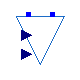OpenIPSL.Electrical.ThreePhase.Loads.Dyn_wye_2Ph_balanced

### Information

This is a two-phase wye load model that allows the user to vary the power consumed during the simulation.

The user needs to input the entire active (P0) and reactive (Q0) powers consumed by the load. Since the model represents a balanced load, the active and reactive powers consumed by each phase are P0/2 and Q0/2. The model allows the load to be represented as constant power only.

In addition to that, the real inputs P_in and Q_in multiply the values of active and reactive power, respectively, being consumed by the load. This allows the load to vary its consumed power over time during the simulation. Note that, since active and reactive powers can vary independently, the load's power factor can also vary. In addition, note that by changing P0 and Q0, the values of power consumed by phases one and two vary identically. This makes the load to be characterized as balanced.

Extends from ThreePhaseComponent (Partial model for inheritance of single-phase base power based on three-phase system base power.).

### Parameters

NameDescription
Power flow data
S_bSystem base [V.A]
S_pPhase base [V.A]
Power flow
P0Initial Active power [W]
Q0Initial Reactive power [var]

### Connectors

NameDescription
P_inexternal P [pu]
Q_inexternal Q [pu]
A
B

##OpenIPSL.Electrical.ThreePhase.Loads.Dyn_wye_2Ph_unbalanced

### Information

This is a two-phase wye load model that allows the user to vary the power consumed during the simulation.

The user needs to input the actives (P0_a and P0_b) and reactives (Q0_a and Q0_b) powers consumed by each phase of the load. Since the values come from independent parameters, the model allows the representation of unbalanced loads. The model allows the load to be represented as constant power only.

In addition to that, the real inputs P_in and Q_in multiply the values of active and reactive power, respectively. This allows the load to vary its consumed power over time during the simulation. Note that, since active and reactive powers can vary independently, the load's power factor can also vary. In addition, note that, since P_in and Q_in have a 2x1 dimension, P0_a, P0_b, Q0_a and Q0_b can vary idependently. This makes the load to be characterized as unbalanced.

Extends from ThreePhaseComponent (Partial model for inheritance of single-phase base power based on three-phase system base power.).

### Parameters

NameDescription
Power flow data
S_bSystem base [V.A]
S_pPhase base [V.A]
Power flow
P0_aInitial Active power [W]
Q0_aInitial Reactive power [var]
P0_bInitial Active power [W]
Q0_bInitial Reactive power [var]

### Connectors

NameDescription
P_inexternal P [pu]
Q_inexternal Q [pu]
A
B

##OpenIPSL.Electrical.ThreePhase.Loads.Dyn_wye_1Ph

### Information

This is a single-phase load model that allows the user to vary the power consumed during the simulation.

The user needs to input the active (P0) and reactive (Q0) powers consumed by the load. The model allows the load to be represented as constant power only.

In addition to that, the real inputs P_in and Q_in multiply the values of active and reactive power, respectively, being consumed by the load. This allows the load to vary its consumed power over time during the simulation. Note that, since active and reactive powers can vary independently, the load's power factor can also vary.

Extends from ThreePhaseComponent (Partial model for inheritance of single-phase base power based on three-phase system base power.).

### Parameters

NameDescription
Power flow data
S_bSystem base [V.A]
S_pPhase base [V.A]
Power flow
P0Initial Active power [W]
Q0Initial Reactive power [var]

### Connectors

NameDescription
P_inexternal P [pu]
Q_inexternal Q [pu]
A
Automatically generated Mon Oct 10 14:35:56 2022.